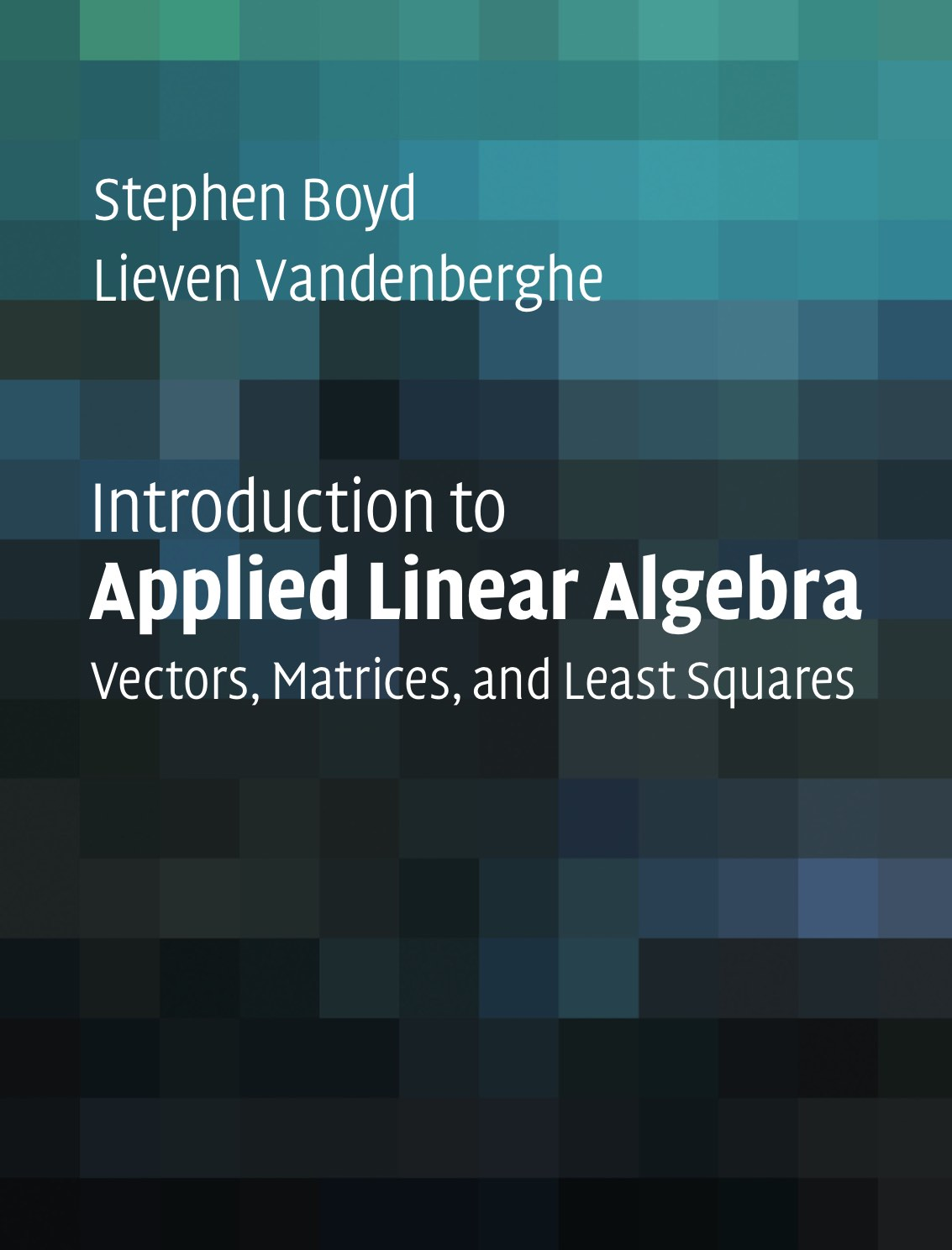# 22659 Applied Linear Algebra: Reading AssignmentsUnless otherwise noted, all reading assignments are from the textbook. We'll be adding to them over the semester.
1. By the end of 1st week (2/19/21): The rank-nullity theorem and LU decomposition from here.

2. By the end of 2nd week (2/26/21): The QR and spectral decompositions from here.

3. By the end of 3rd week (3/5/21): Matrix diagonalization and singular value decomposition from here.

4. By the end of 4th week (3/12/21): Vectors, linear functions, norm and distance, linear independence, and matrices.

5. By the end of 5th week (3/19/21): Introduction to the Julia language from here.

6. By the end of 6th week (3/26/21): Nowruz holiday

7. By the end of 7th week (4/2/21): Nowruz holiday

8. By the end of 8th week (4/9/21): Clustering and matrix examples.

9. By the end of 9th week (4/16/21): Linear equations and linear dynamical systems.

10. By the end of 10th week (4/23/21): Matrix multiplication and matrix inverses.

11. By the end of 11th week (4/30/21): Least squares.

12. By the end of 12th week (5/7/21): Least squares data fitting.

13. By the end of 13th week (5/14/21): Least squares classification.

14. By the end of 14th week (5/21/21): Multi-objective least squares.

15. By the end of 15th week (5/28/21): Constrained least squares.

16. By the end of 16th week (6/4/21): Constrained least squares applications.

17. By the end of 17th week (6/11/21): Nonlinear least squares.

18. By the end of 18th week (6/18/21): Constrained nonlinear least squares.